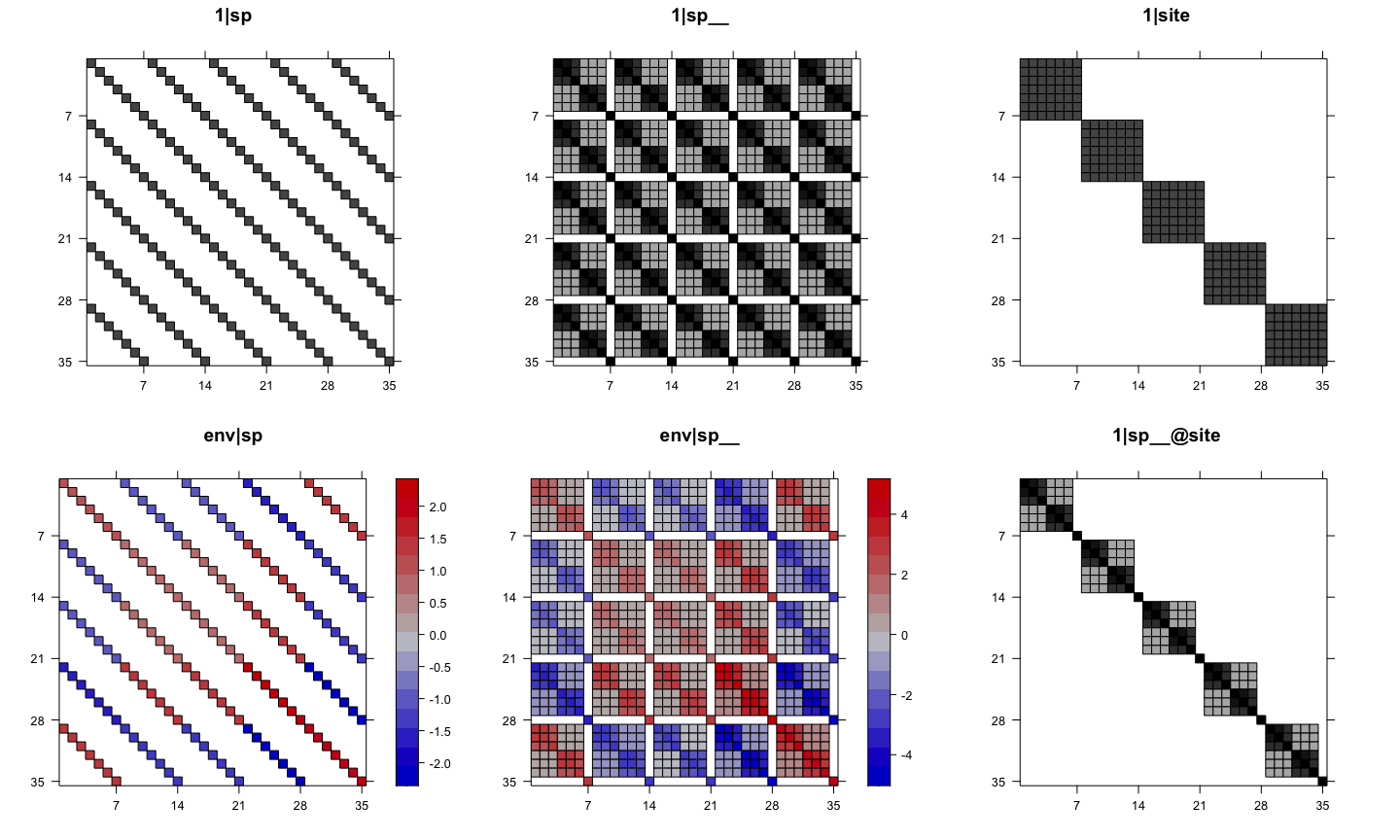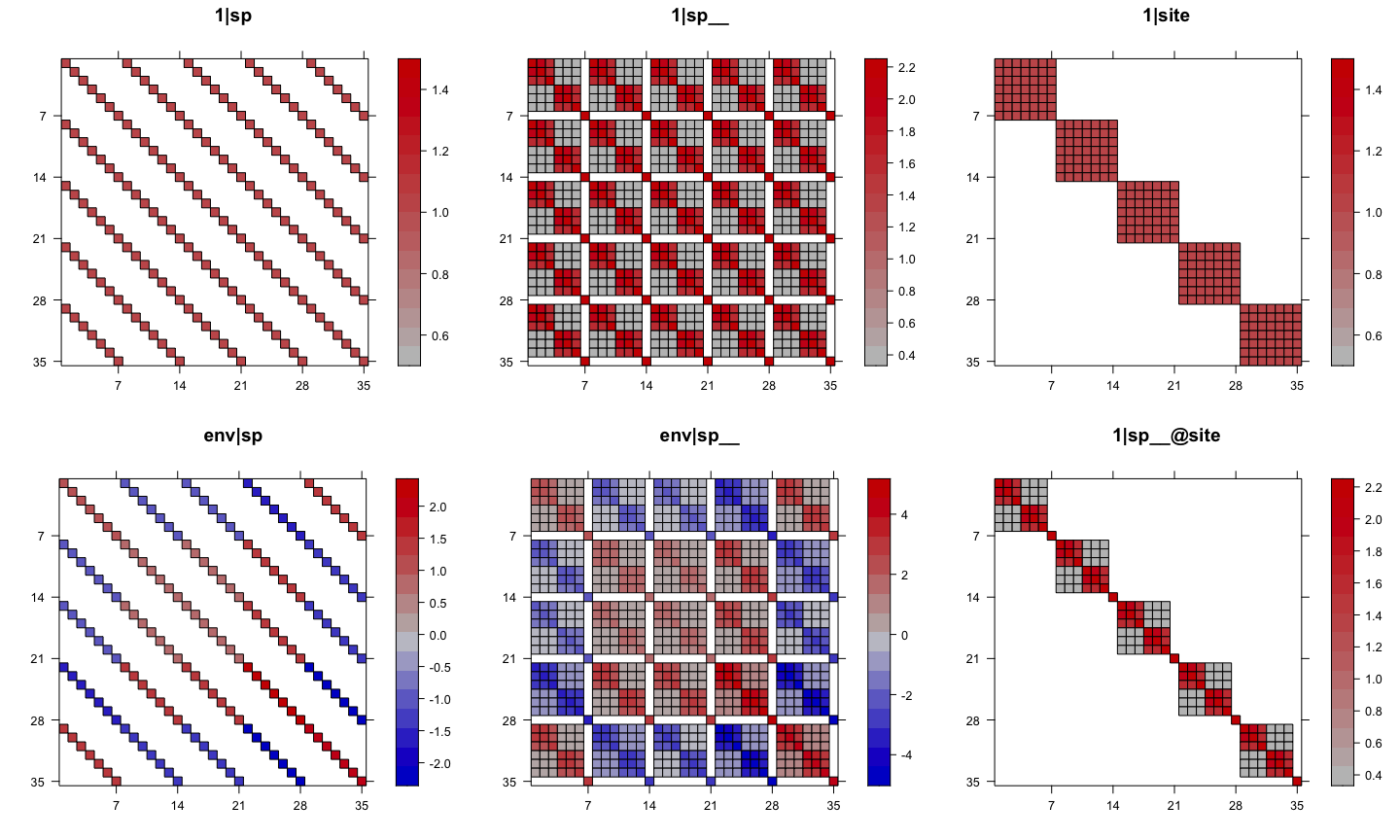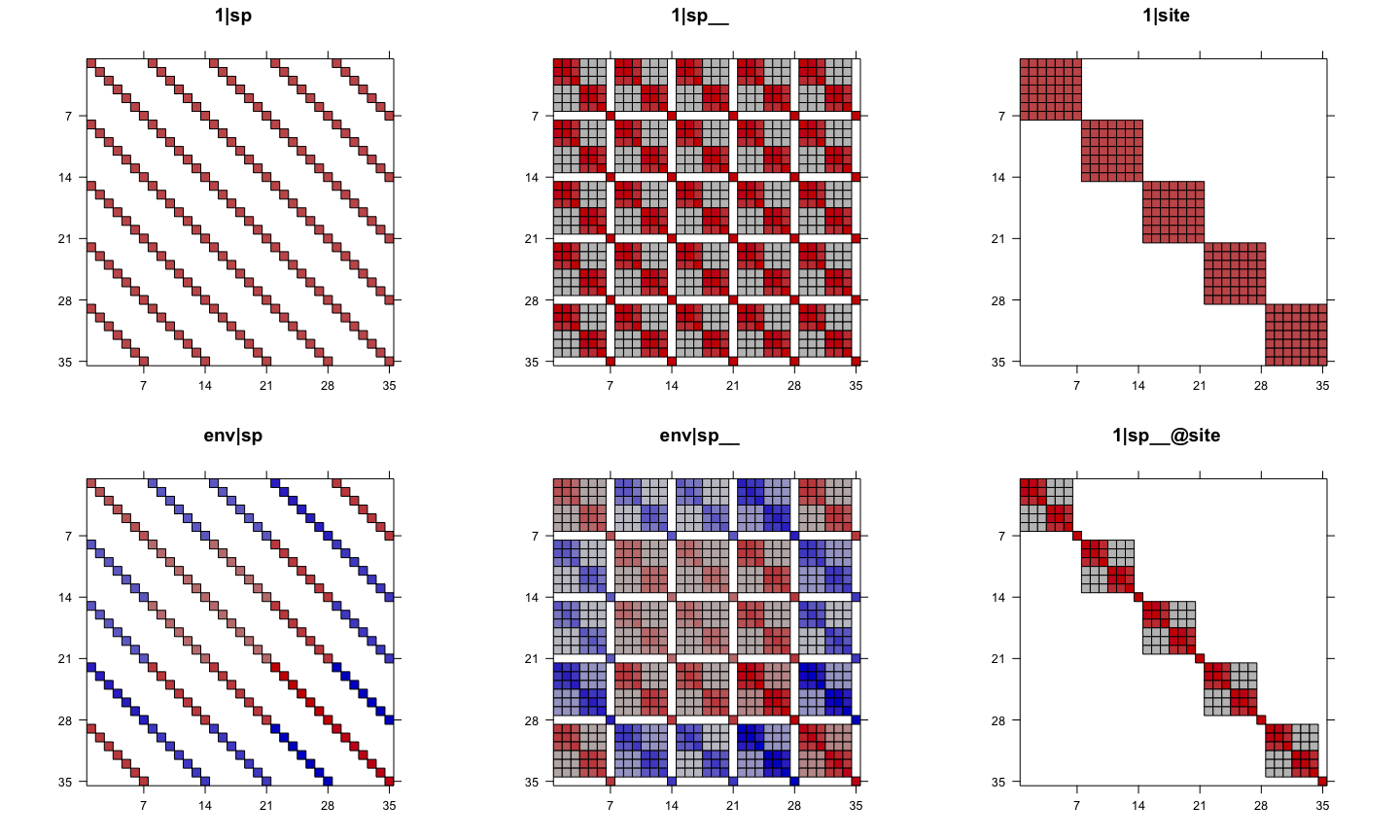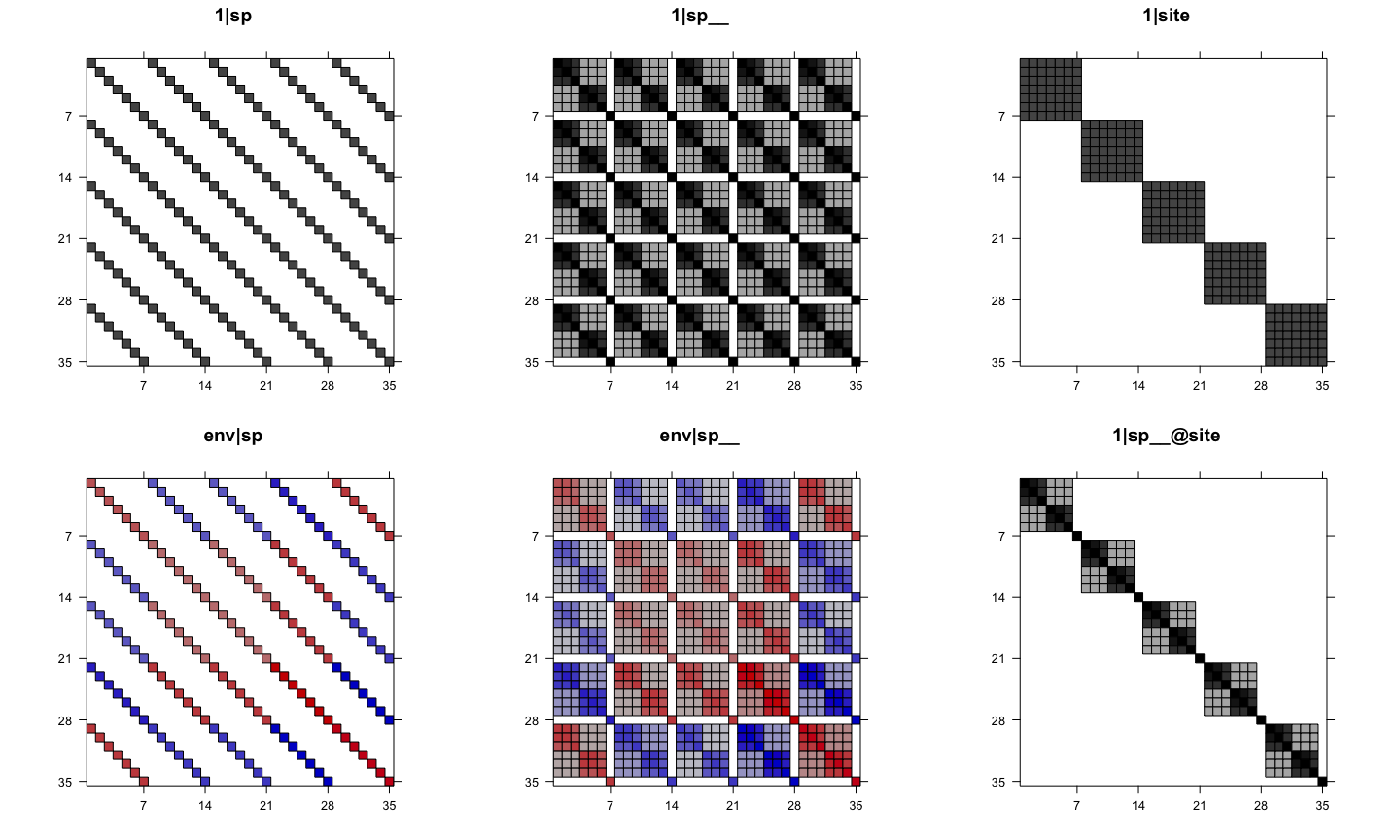# Plot random terms of communityPGLMM

#### 2020-12-17

This vignette will show how to visualize the var-covariance matrix of random terms for `communityPGLMM` models.

# Main function

The main function to use is `phyr::pglmm_plot_re()` (alias: `phyr::pglmm_plot_ranef()`, `phyr::communityPGLMM.show.re()`, `phyr::communityPGLMM.plot.re()`). Here are the arguments of this function:

``````args(phyr::pglmm_plot_re)
## function (formula = NULL, data = NULL, family = "gaussian", sp.var = "sp",
##     site.var = "site", tree = NULL, tree_site = NULL, repulsion = FALSE,
##     x = NULL, show.image = TRUE, show.sim.image = FALSE, random.effects = NULL,
##     tree.panel.space = 0.5, title.space = 5, tree.size = 3, ...)
## NULL``````

Some brief explanation of arguments:

• `x`: a model with class communityPGLMM, if it is specified, then all other argument before x will be ignored.
• `show.image` (`TRUE` or `FALSE`): whether to plot the var-cov matrix of random terms?
• `show.sim.image` (`TRUE` or `FALSE`): whether to plot simulated site by species matrix for all random terms?
• `add.tree.sp` (`TRUE` or `FALSE`): when `show.sim.image = TRUE`, whether to add a phylogeny of species at the top of each matrix plot?
• `add.tree.site` (`TRUE` or `FALSE`): when `show.sim.image = TRUE`, whether to add a phylogeny of sites at the right of each matrix plot? This can be useful for bipartite problems (e.g. pollinators (species) and plants (sites)).
• `tree.size` (default is 3): the height of the phylogenies to plot, unit is number of lines.

This function will return a hidden list, which includes all the var-cov matrices of random terms, simulated site by species matrices, individual plots, and all plots in one figure for both var-cov matrices and simulated ones. Therefore, we can extract specific plots and then update them or generate new figure with `gridExtra::grid.arrange()`. This is because all generated plots are based on `lattice` package and are all `grid` object. Therefore, we can also use `gridExtra::arrangeGrob()` to put multiple plots in one figure and then use `ggplot2::ggsave()` to save it as external file (e.g. PDF). Of course, `pdf()` and `dev.off()` will also work.

# Simulate data

Now, let’s show how to use this function to help us understanding better the random terms.

``````library(ape)
library(phyr)
suppressPackageStartupMessages(library(dplyr))

set.seed(12345)
nspp <- 7
nsite <- 5
# Simulate a phylogeny that has a lot of phylogenetic signal (power = 1.3)
phy <- compute.brlen(rtree(n = nspp), method = "Grafen", power = 1.3)
# Simulate species means
sd.sp <- 1
mean.sp <- rTraitCont(phy, model = "BM", sigma = sd.sp^2)
Y.sp <- rep(mean.sp, times = nsite)
# Phylogenetically correlated response of species to env
sd.trait <- 1
trait <- rTraitCont(phy, model = "BM", sigma = sd.trait)
trait <- rep(trait, times = nsite)
# Simulate site means
sd.site <- 1
mean.site <- rnorm(nsite, sd = sd.site)
Y.site <- rep(mean.site, each = nspp)
# Site-specific environmental variation
sd.env <- 1
env <- rnorm(nsite, sd = sd.env)
# Generate covariance matrix for phylogenetic attraction
sd.attract <- 1
Vphy <- vcv(phy)
Vphy <- Vphy / (det(Vphy) ^ (1 / nspp))
V.attract <- kronecker(diag(nrow = nsite, ncol = nsite), Vphy)
Y.attract <- array(t(mvtnorm::rmvnorm(n = 1, sigma = sd.attract ^ 2 * V.attract)))
# Residual errors
sd.e <- 1
Y.e <- rnorm(nspp * nsite, sd = sd.e)
# Construct the dataset
d <- data.frame(sp = rep(phy\$tip.label, times = nsite),
site = rep(1:nsite, each = nspp),
env = rep(env, each = nspp))
# Simulate abundance data
d\$Y <- Y.sp + Y.attract + trait * d\$env + Y.e
##   sp site       env          Y
## 1 t4    1 -1.060266 -1.3475684
## 2 t2    1 -1.060266  1.2422030
## 3 t5    1 -1.060266 -1.2711509
## 4 t3    1 -1.060266  1.8940820
## 5 t6    1 -1.060266  1.5771805
## 6 t7    1 -1.060266  0.3308875

# fit a model
mod_1 = pglmm(Y ~ 1 + env + (1|sp__) + (1|site) + (env|sp__) + (1|sp__@site),
data = d, cov_ranef = list(sp = phy))
summary(mod_1)
## Linear mixed model fit by restricted maximum likelihood
##
## Call:Y ~ 1 + env
##
## logLik    AIC    BIC
## -64.34 146.68 150.38
##
## Random effects:
##              Variance   Std.Dev
## 1|sp        2.085e-06 0.0014439
## 1|sp__      4.218e-01 0.6494991
## 1|site      1.235e-07 0.0003515
## env|sp      1.209e-06 0.0010993
## env|sp__    5.434e-01 0.7371383
## 1|sp__@site 1.375e-01 0.3707606
## residual    1.885e+00 1.3729463
##
## Fixed effects:
##               Value Std.Error Zscore Pvalue
## (Intercept) 0.83092   0.63742 1.3036 0.1924
## env         0.75052   0.68819 1.0906 0.2755``````

# Var-cov matrices of random terms

## Plot var-cov matrices of all random terms in one figure

``````# plot var-cov matrices of random terms
mod1re = pglmm_plot_re(Y ~ 1 + env + (1|sp__) + (1|site) + (env|sp__) + (1|sp__@site),
data = d, cov_ranef = list(sp = phy), show.image = TRUE,
show.sim.image = FALSE)``````In the above plot, we can see that some of the panels are black-white but some have colors. This is because, by default, if a matrix has both positive and negative values, then the function will use red-blue color and will draw a key for that (use `colorkey = FALSE` to suppress it). If a matrix does not have negative values, then the function will use black/white color (use `useAbs = FALSE` to use color instead, and use `colorkey = FALSE` to suppress key if wanted). In both cases, value 0 will be white so that the structure of the var-cov matrix can be easier to see.

``````# all use color with useAbs = FALSE
pglmm_plot_re(Y ~ 1 + env + (1|sp__) + (1|site) + (env|sp__) + (1|sp__@site),
data = d, cov_ranef = list(sp = phy), show.image = TRUE,
show.sim.image = FALSE, useAbs = FALSE)``````For the above plot, notice that for `1|sp` and `1|site`, all values are either 1 or 0 even though we have a range in the key. We can suppress the key with `colorkey = FALSE`.

``````# suppress key with colorkey = FALSE
pglmm_plot_re(Y ~ 1 + env + (1|sp__) + (1|site) + (env|sp__) + (1|sp__@site),
data = d, cov_ranef = list(sp = phy), show.image = TRUE,
show.sim.image = FALSE, useAbs = FALSE, colorkey = FALSE)``````We can also just use `colorkey = FALSE` and still use black/white color for matrices that do not have negative values (without setting `useAbs`).

``````# suppress colorkey, let the function decide whether use color or not
pglmm_plot_re(Y ~ 1 + env + (1|sp__) + (1|site) + (env|sp__) + (1|sp__@site),
data = d, cov_ranef = list(sp = phy), show.image = TRUE,
show.sim.image = FALSE, colorkey = FALSE)``````To make all plots black or white, use `useAbs = TRUE`.

``````# all black and white
pglmm_plot_re(Y ~ 1 + env + (1|sp__) + (1|site) + (env|sp__) + (1|sp__@site),
data = d, cov_ranef = list(sp = phy), show.image = TRUE,
show.sim.image = FALSE, useAbs = TRUE)``````